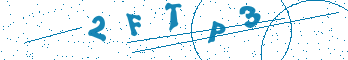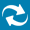# Error: COMP96_0071: Operator "<operator>" is not defined for such operands

## Description

COMP96_0071 is caused by using the undefined %s operator.

```entity en is
end;

architecture ar of en is
signal a : integer;
signal c : bit_vector(3 downto 0);
signal d : bit_vector(3 downto 0);
begin
d<= a+c; --COMP96_0071
end;
```

## Solution

Please do one of the following:

• Declare the + operator for the bit and boolean types.

```entity en is
end;

architecture ar of en is
signal a : integer;
signal c : bit_vector(3 downto 0);
signal d : integer;
function "+"(l:integer;r:bit_vector) return integer is
variable i : integer;
begin
--(...)
return i;
end function;
begin
d<= a+c;
end;
```
• Convert the type operator to a type where the operator is defined.

```entity en is
end;

architecture ar of en is
signal a : integer;
signal c : bit_vector(3 downto 0);
signal d : integer;
function conv_func (a:bit_vector) return integer is
variable i : integer;
begin
--()
return i;
end function;
begin
d<= a+conv_func(c);
end;
```
• Use the conversion function or the operator from one of the predefined libraries.

```library ieee;
use ieee.numeric_bit.all;
entity en is
end;

architecture ar of en is
signal a : integer;
signal c : bit_vector(3 downto 0);
signal d : integer;
begin
d<= a+to_integer(unsigned(c));
end;
```